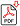Search for Articles:

## Application of Numerical Methods in Transient Analysis

Otoo Henry, Buabeng Albert, Inkoom Justice

Abstract: Transient analysis of an RLC circuit (or LCR circuit) comprising of a resistor, an inductor, and a capacitor are analysed using the Heun?s and the Runge-Kutta 4th order methods. Kirchhoff?s voltage and current laws were used to generate equations for voltages and currents across the elements in an RLC circuit. From Kirchhoff?s law, the second order differential equations were later transformed into first order differential equations by substitution. The numerical methods were then used together with MATLAB simulations to check how changes in resistance affects transient. Errors associated with selected numerical methods were then measured with Big ?O? notation (truncation). From the study, it was observed that, the computational values of the Heun?s method converged faster than that of Runge-Kutta 4th order method. However, the errors incurred in Runge-Kutta 4th order method were very minimal as compared to that of Heun?s method, thus, Runge-Kutta 4th order method was concluded to be more accurate than Heun?s method.

Keywords: RLC Circuit, Numerical Methods, Big ?O? Notation, MATLAB Simulations

Country: Ghana, Subject Area: Mathematics

Pages: 2108 - 2112

Edition: Volume 8 Issue 5, May 2019